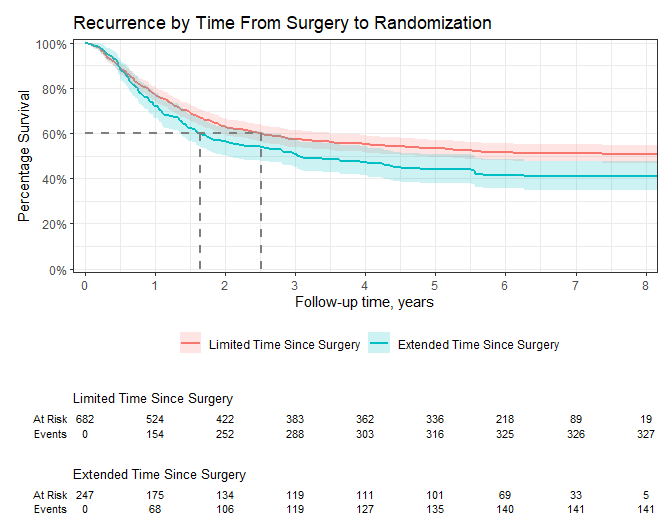## Introduction

The {ggsurvfit} package eases the creation of time-to-event (aka survival) endpoint figures with ggplot. The concise and modular code creates images that are ready for publication or sharing.

## Why ggsurvfit?

• Raw variable names do not appear in the figure legend, e.g. "sex=Female".

• You can modify the x-axis scales and the risk table will still align with the plot.

• Each add-on function (e.g. add_confidence_interval(), etc.) is written as a proper ggplot2 ‘geom’, meaning that the package functions can be woven with ggplot2 functions seamlessly.

## Installation

You can install the development version of ggsurvfit from GitHub with:

# install.packages("devtools")
devtools::install_github("ddsjoberg/ggsurvfit")

## Examples

Let’s begin with an example illustrating a common Kaplan-Meier survival curve.

It’s recommended to use the survfit2() function with this package, rather than survival::survfit() to ensure all quantities are able to be calculated from any environment the functions are called.

library(ggsurvfit)
library(ggplot2)

p <-
survfit2(Surv(time, status) ~ sex, data = df_lung) |>
ggsurvfit(size = 1) +
add_quantile(color = "gray40", size = 1)
pBecause each of the functions are written as proper ggplot geoms, you can add any ggplot function to modify the figure.

p +
labs(
y = "Probability of survival",
x = "Months since treatment",
title = "Kaplan-Meier Estimate of Survival by Sex",
# remove the fill and color legend labels (Sex appears in title)
fill = NULL, color = NULL
) +
# reduce padding on edges of figure, and format axes
scale_y_continuous(label = scales::percent, expand = c(0.01, 0)) +
scale_x_continuous(breaks = 0:5*6, expand = c(0.02, 0))The package also supports unstratified models and you can add multiple quantile guidelines.

survfit2(Surv(time, status) ~ 1, data = df_lung) |>
ggsurvfit(size = 1) +
add_quantile(linetype = 3, size = 1) +
add_quantile(y_value = 0.25, linetype = 2, size = 1) +
add_risktable()You can even facet…but you cannot facet with the risktable, however.

survfit2(Surv(time, status) ~ sex, data = df_lung) |>
ggsurvfit(size = 1) +
add_quantile(linetype = 3, size = 1) +
ggplot2::facet_grid(~strata)The package works seamlessly with other ggplot extension package, such as {gghighlight}.

survfit2(Surv(time, status) ~ ph.ecog, data = df_lung) |>
ggsurvfit(size = 1) +
ggplot2::labs(color = "Gender") +
gghighlight::gghighlight(strata == "Asymptomatic", calculate_per_facet = TRUE)The package also plots cumulative incidence estimates in the presence of competing events.

library(tidycmprsk)

cuminc(Surv(ttdeath, death_cr) ~ trt, trial) %>%
ggcuminc(outcome = "death from cancer", size = 1) +
scale_x_continuous(breaks = 0:4 * 6, expand = c(0.02, 0))